×#### Thank you for registering.

One of our academic counsellors will contact you within 1 working day.

Click to Chat

1800-1023-196

+91-120-4616500

CART 0

• 0

MY CART (5)

Use Coupon: CART20 and get 20% off on all online Study Material

ITEM
DETAILS
MRP
DISCOUNT
FINAL PRICE
Total Price: Rs.

There are no items in this cart.
Continue Shopping• Complete JEE Main/Advanced Course and Test Series
• OFFERED PRICE: Rs. 15,900
• View Details

```Chapter 4: Rational Numbers Exercise – 4.2

Question: 1

Express each of the following as rational number with positive denominator:

(i)  (-15)/(-28)

(ii)  6/(-9)

(iii)   (-28)/(-11)

(iv)   19/(- 7)

Solution:

Rational number with positive denominators:

(i) Multiplying the number by -1, we get: -15 – 28 = -15 x -1 - 28 x – 1 = 1528

(ii) Multiplying the number by -1, we get:  6 – 9 = 6x – 1 - 9x – 1 = - 69

(iii) Multiplying the number by -1, we get: – 28 – 11= – 28 x – 1 – 11 x – 1 = 2811

(iv) Multiplying the number by -1, we get: 19 – 7 = 19 x – 1 - 7 x - 1= -197

Question: 2

Express 3/5 as a rational number with numerator:

(i)  6

(ii) -15

(iii)  21

(iv) – 27

Solution:

Rational number with numerator:

(i) 6 is: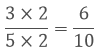(Multiplying numerator and denominator by 2)

(ii) -15 is:(Multiplying numerator and denominator by - 5)

(iii) 21 is:(Multiplying numerator and denominator by 7)

(iv) - 27 is: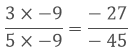(Multiplying numerator and denominator by - 9)

Question: 3

Express 5/7 as a rational number with denominator:

(i) -14

(ii) 70

(iii) -28

(iv) - 84

Solution:

5/7 as a rational number with denominator:

(1) -14 is:(Multiplying numerator and denominator by -2)

(ii) 70 is:(Multiplying numerator and denominator by 10)

(iii) - 28 is: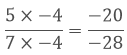(Multiplying numerator and denominator by - 4)

(iv) - 84 is: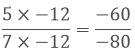(Multiplying numerator and denominator by -12)

Question: 4

Express 3/4 as a rational number with denominator:

(i) 20

(ii) 36

(iii) 44

(iv) - 80

Solution:

3/4 as rational number with denominator:

(i) 20 is:(Multiplying numerator and denominator by 5)

(ii) 36 is: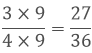(Multiplying numerator and denominator by 9)

(iii)  44 is: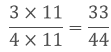(Multiplying numerator and denominator by 11)

(iv) - 80 is: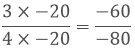(Multiplying numerator and denominator by - 20)

Question: 5

Express 2/5 as a rational number with numerator:

(i) -56

(ii) 154

(iii) -750

(iv)  - 80

Solution:

2/5 as a rational number with numerator:

(i)  - 56 is: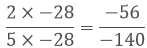(Multiplying numerator and denominator by -28)

(ii) 154 is: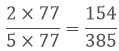(Multiplying numerator and denominator by 77)

(iii) – 750 is: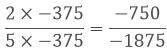(Multiplying numerator and denominator by -375)

(iv) 500 is: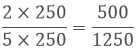(Multiplying numerator and denominator by 250)

Question: 6

Express (-192)/108 as a rational number with numerator:

(i) 64

(ii) -16

(iii) 32

(iv) - 48

Solution:

Rational number with numerator:

(i)  64 as numerator: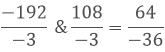(Dividing the numerator and denomintor by -3)

(ii) -16 as numerator: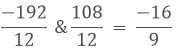(Dividing the numerator and denomintor by 12)

(iii) 32 as numerator: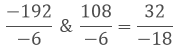(Dividing the numerator and denomintor by - 6)

(iv) - 48 as numerator:(Dividing the numerator and denomintor by 4)

Question: 7

Express  168/(-294)  as a rational number with denominator:

(i) 14

(ii) -7

(iii) - 49

(iv) 1470

Solution:

Rational number with denominator:

(i) 14 as denominator:(Dividing the numerator and denomintor by -21)

(ii) -7 as denominator: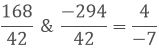(Dividing the numerator and denomintor by 42)

(iii) - 49 as denominator: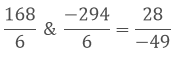(Dividing the numerator and denomintor by 6)

(iv) 1470 as denominator: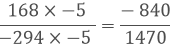(Multiplying the numerator and denomintor by – 5)

Question: 8

Write (-14)/42 form so that numerator is equal to:

(i) -2

(ii)  7

(iii) 42

(iv) -70

Solution:

Rational number with numerator:

(i) -2 is: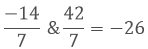(Dividing numerator and denominator by 7)

(ii) 7 is: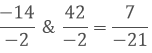(Dividing numerator and denominator by -2)

(iii) 42 is: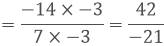(Multiplying numerator and denominator by -3)

(iv) -70 is: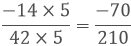(Multiplying numerator and denominator by 5)

Question: 9

Select those rational numbers which can be written as a rational number with numerator 6: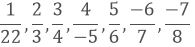Solution:

Given rational numbers that can be written as a rational number with numerator 6 are:

1/22 (On multiplying by 6) = 6/132, 2/3 (On multiplying by 3)

= 6/9, 3/4 (On multiplying by 2)

= 6/8, (-6)/7 (On multiplying by -1)

= 6/(-7)

Question: 10

Select those rational numbers which can be written as a rational number with denominator 4:Solution:

Given rational numbers that can be written as a rational number with denominator 4 are:

7/8 (On dividing by 2) = 3.5/4,

64/16 (On dividing by 4) = 16/4,

36/-12(On dividing by 3) = 12/-4 = -12/4,

16/17 can’t be expressed with a denominator 4.

5/- 4(On multiplying by -1) = - 5/4

140/28(On dividing by 7) = 20/4

Question: 11

In each of the following, find an equivalent form of the rational number having

common denominator:

(i)  3/4 and 5/12

(ii)   2/3, 7/6 and 11/12

(iii)   5/7, 3/8, 9/14 and 20/21<

Solution:

Equivalent forms of the rational number having common denominator are:

(i) 3/4 = (3 × 3)/(4 × 3) = 9/12 and 512.

(ii) 2/3 = (2 × 4)/(3 × 4) = 8/12 and 7/6 = (7×2)/(6×2) = 14/12 and 11/12

Forms are 8/12, 14/12 and 11/12

(iii) 5/7 = (5 × 24)/(7 × 24) = 120/168, 3/8 = (3 × 21)/(8 × 21) = 63/168, 9/14

= (9 × 12)/(14 × 12)

= 108/168 and 20/21

= (20 × 8)/(21×8)

=160/168

Forms are 120/168, 63/168, 108/168 and 160/168.
```### Course Features

• 728 Video Lectures
• Revision Notes
• Previous Year Papers
• Mind Map
• Study Planner
• NCERT Solutions
• Discussion Forum
• Test paper with Video Solution